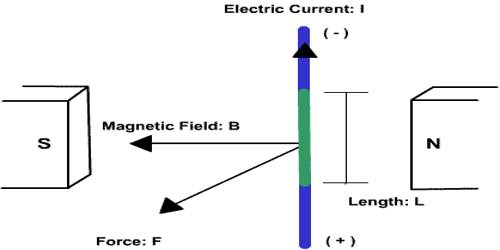# Lorentz Force

Lorentz force: The force that a charge experiences while moving simultaneously through the electric field and magnetic field is called Lorentz force.

The force exerted on a charged particle q moving with velocity v through an electric E and magnetic field B. The entire electromagnetic force F on the charged particle is called the Lorentz force and is given by F = qE + qv × B.The implications of this expression include:

1. The force is perpendicular to both the velocity v of the charge q and the magnetic field B.
2. The magnitude of the force is F = qvB sinθ where θ is the angle < 180 degrees between the velocity and the magnetic field. This implies that the magnetic force on a stationary charge or a charge moving parallel to the magnetic field is zero.
3. The direction of the force is given by the right-hand rule. The forced relationship above is in the form of a vector product.